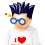Support us .Net Basics C# SQL ASP.NET Aarvi MVC Slides C# Programs Subscribe Download

### Part 1 - Can you store different types in an array in c#

Can you store different types in an array in c#?
Yes, if you create an object array. Here is an example. Since all types inherit (directly or indirectly) from object type, we can add any type to the object array, including complex types like Customer, Employee etc. You need to override the ToString() method if you want meaningful output when ToString() method is invoked.

class Program
{
static void Main()
{
object[] objectArray = new object;
objectArray = 101; // integer
objectArray = "C#"; // string

Customer c = new Customer();
c.ID = 99;
c.Name = "Pragim";

objectArray = c; // Customer - Complext Type

// loop thru the array and retrieve the items
foreach (object obj in objectArray)
{
Console.WriteLine(obj);
}
}
}

class Customer
{
public int ID { get; set; }
public string Name { get; set; }

public override string ToString()
{
return this.Name;
}
}

Another alternative is to use ArrayList class that is present in System.Collections namespace.
class Program
{
static void Main()
{
System.Collections.ArrayList arrayList = new System.Collections.ArrayList();

Customer c = new Customer();
c.ID = 99;
c.Name = "Pragim";

arrayList.Add(c); // Customer - Complext Type

// loop thru the array and retrieve the items
foreach (object obj in arrayList)
{
Console.WriteLine(obj);
}
}
}1.Questions faced in an interview: Problem1:
You are given any array of length n,containing both +ve & -ve numbers.
You need to write a program to find the two elements such that their sum is closest to zero.
eg.
Input array[15,5,-20,30,-45]
Output should be 15,-20 as their sum is 5 which is closest to zero.

Problem2:
You are given any array of length n.You have to sort the array elements in decreasing order of their frequency.Use of Collections is not allowed.Write a function which sorts the element accordingly.
eg.
Input :[2,3,4,2,8,1,1,2]
Output:[2,2,2,1,1,3,4,8]
Can u record a video for it.?Thanks in advance :)﻿

1.int[] intArray = new int { 8, 10, 2, 6, 3 };
Array.Sort(intArray);

Using foreach to loop value

2.This comment has been removed by the author.

3.//Indexes of output elements
int i1 = 0, i2 = 0;
//
int k = 100;
for (int i = 0; i < input.Length - 1; i++ )
{
for (int j = i + 1; j < input.Length; j++)
{
if (Math.Abs( input[i] + input[j]) < k)
{
k = Math.Abs (input[i] + input[j]);
i1 = i;
i2 = j;
}
}
}

It would be great if you can help share these free resources